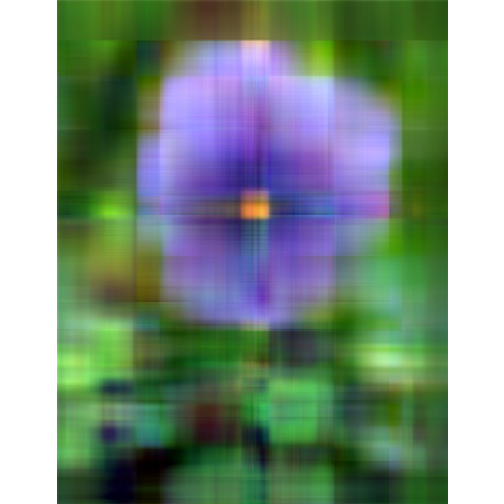Singular Value Decomposition and Image Processing

The singular value decomposition (SVD) is a factorization of a real or complex matrix. It has many useful applications in signal processing and statistics.

Singular Value Decomposition

SVD is the factorization of a $$m \times n$$ matrix $$Y$$ into three matrices as:

$$\mathbf{Y = UDV^\top}$$

With:

• $$U$$ is an $$m\times n$$ orthogonal matrix
• $$V$$ is an $$n\times n$$ orthogonal matrix
• $$D$$ is an $$n\times n$$ diagonal matrix

In R The result of svd(X) is actually a list of three components named d, u and v, such that Y = U %*% D %*% t(V).

Example

• we can reconstruct Y

Image processing

• Load the image and convert it to a greyscale:• Apply SVD to get U, V, and D
• Plot the magnitude of the singular valuesNot that, the total of the first n singular values divided by the sum of all the singular values is the percentage of "information" that those singular values contain. If we want to keep 90% of the information, we just need to compute sums of singular values until we reach 90% of the sum, and discard the rest of the singular values.Image Compression with the SVD

Here we continue to show how the SVD can be used for image compression (as we have seen above).

• Original image• Singluar Value k = 1• Singluar Value k = 5• Singluar Value k = 20• Singluar Value k = 50• Singluar Value k = 100• Analysis

With only 10% of the real data we are able to create a very good approximation of the real data.

References

If you liked this post, you can share it with your followers or follow me on Twitter!